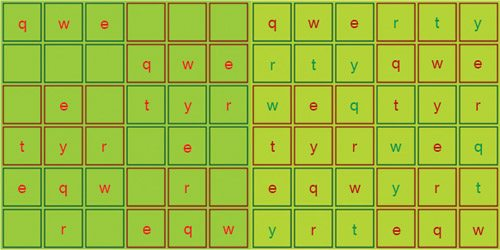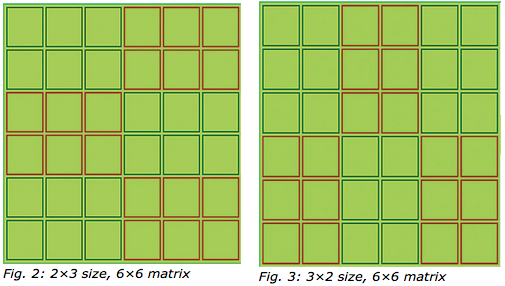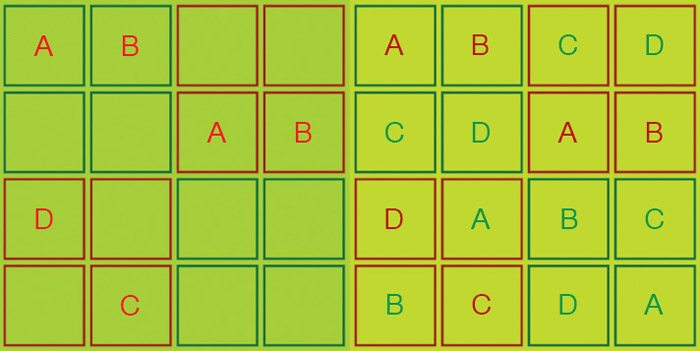# JavaScript-based Multiformat Sudoku Puzzle Solver

Bukya Sridhar

4116

Sudoku is a mathematical-cum-logical puzzle where every digit should come uniquely in every row and column without repetition. We generally find Sudoku puzzles in dailies and magazines with various levels of toughness with respect to placement of numbers, number of puzzle digits and blanks.

Mostly, dailies publish only numerical puzzles with limited matrix length of either 9×9 or 6×6. In this project, we show that non-numeric entities can also be used to form a Sudoku grid. One can also define the size of the matrix beyond 6×6 and 9×9. In this project one can use numeric digits from 1-9, and alphabets in upper and lower cases (A-Z, a-z).Fig. 1: 6×6 matrix with non-numerics (unsolved and solved)Fig. 4: 4×4 matrix with unsolved and solved puzzle

The 6×6 matrix with non-numerics is shown in Fig. 1. Likewise, one can have a one-cell grid of 1×1 size to 2401-celled grid of 7×7 size with the above characters.

There are two programs for this project: sudo_alpha.html and sudo_num.html. The first code is for solving the alphabet-based Sudoku puzzle and the later code is for numeric-based Sudoku puzzle.

In both case, the default size of the matrix is 3×3, which is a 9×9 matrix having 81-cell grids. User can change the size from 1×1 up to 7×7 as per requirement.

The program